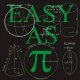Feeds:
Posts

## SQL SERVER – How to sum a varchar column

We usually see this kind of problem in a legacy system wherein the datatype of a table’s column is something but the data stored in it belongs to some different datatypes. And most of the time these datatypes are compatible with each other so it does not create any issues at the time of insertion. But, when it comes to data manipulation you feel handicapped. However, you can resolve these issues using some scripting. But you must remember that before doing any manipulation you must know the actual data is in which datatype (small integer, integer, bigint, numeric, decimal, money, float) and according to that, you need to convert and then manipulate it.

Lets resolve this issue step by step :

Step 1 :
Let me create a table to demonstrate the solution.

```USE tempdb
GO
CREATE TABLE tbl_sample
(
[ID] INT,
[Column_varchar] VARCHAR(10)
)
GO
```

Step 2 :
Insert some dummy data to perform aggregate SUM on column ([Column_varchar]). Given below is the script.

```USE tempdb
GO
INSERT INTO tbl_sample VALUES (1,12345)
INSERT INTO tbl_sample VALUES (2,1.234)
INSERT INTO tbl_sample VALUES (3,'a64')
INSERT INTO tbl_sample VALUES (4,'1,200')
```

Step 3 :
Browse the data from the table and check the datatypes. Given below is the script.

```USE tempdb
GO
SELECT * FROM tbl_sample
```Step 4 :
As you can see there is a ‘,’ (Comma) in ID no 4 in the table. If you find cases like this, you need to use money datatype to convert.
Given below is the script to convert it.

```USE tempdb
GO
SELECT
(CASE WHEN ISNUMERIC([Column_varchar])=1
THEN CONVERT(MONEY
,[Column_varchar]) ELSE 0 END)
AS [Converted to Numeric]
FROM tbl_sample
GO
```Step 5 :
Once you convert it into any number datatype after that just perform any aggregate function on it. Lets SUM the column ([column varchar]) in the table (tbl_sample).
Given below is the script.

```SELECT
SUM((CASE WHEN ISNUMERIC([Column_varchar])=1
THEN CONVERT(MONEY,[Column_varchar]) ELSE 0 END)
)
AS [Converted to Numeric]
FROM tbl_sample
GO
```### 4 Responses

1. on June 7, 2013 at 1:27 am | ReplyAndrew Judd

If you are using SQL Server 2012, then you can use the TRY_PARSE function to sum it up.

SELECT
SUM(TRY_PARSE([Column_varchar] AS MONEY))
AS [Converted to Numeric]
FROM tbl_sample
GO

It will return NULL if the data cannot be cast.

• on June 9, 2013 at 12:34 am | ReplyMuhammad Imran

Hi Andrew,

Very nice solution. Thank you.

Imran

2. on October 17, 2014 at 4:56 pm | ReplySatya

select SUM(CAST(REPLACE(Hours, ‘:’,’.’) AS float)) as THours FROM tbl_EmpTimeSheet

3. on October 31, 2014 at 6:43 pm | Replysgnanasekaranbe

thank you very usefull